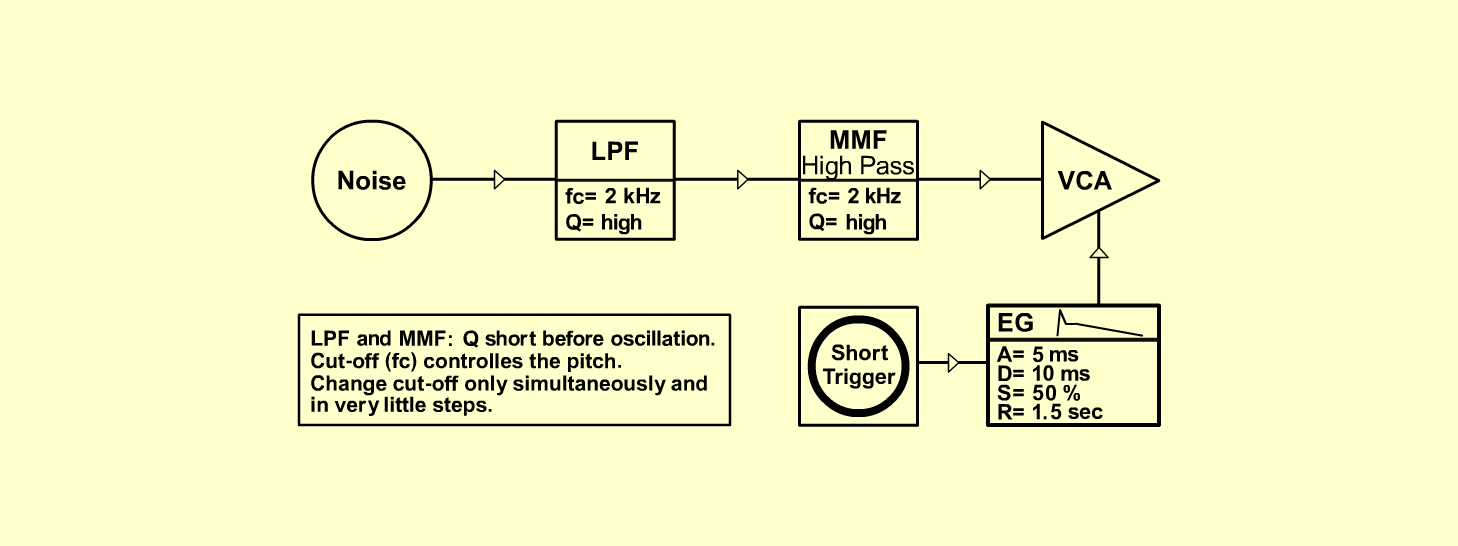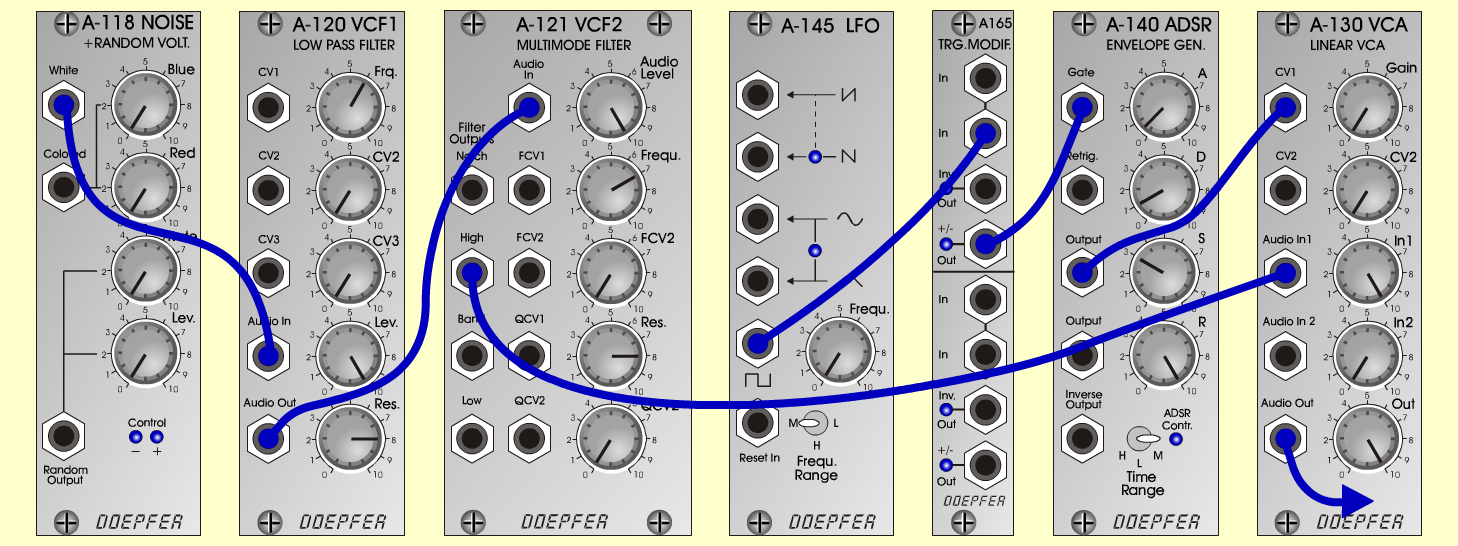SphereFXD o e p f e r A - 100 Connections: Settings: A-118 (White)  <=>  A-120 (Audio In)   A-120 (Audio Out)  <=>  A-121 (Audio In)   A-121 (High)  <=>  A-131 (Audio In 1)   A-140 (Output)  <=>  A-131 (CV 1)   A-131 (Audio Out)  <=> Amplifier    A very short trigger : KB, Seq or   A-145 (Pulse)  <=>  A-165 (In)   A-165 (Out)  <=>  A-140 (Gate) A-120 (Freq = 6, Lev = 10, Res = 8)   A-121 (Audio Level = 10, Freq = 7, Res =8)   A-140 (A = 0.5, D = 1, S = 3, R = 10, Range = M)   A-145 (Frq = 0, Range = M)   A-130 (Gain = 0, Audio In 1 = 10, Audio Out = 10) Notes: Eliminate the click from ADSR-Attack with   values lower or higher than 0.5.   Set Q from A-120 and A-121 short before   oscillation.   Cut-off (fc) controlles the pitch. Change cut-off   only simultaneously and in very little steps.   The sound moves from left to right with   A-140/2 (Output)  <=>  A-134 (Audio In 1)   A-134 (Left / Right Output)  <=>  Amplifier   (Left / Right Input)   A-140 (A = 1, D = 1.5, S = 0, R = 7, Range =M)   A-134 (Pan = 2, CV 2 = 10) Josef MuellerSound sample Sphere